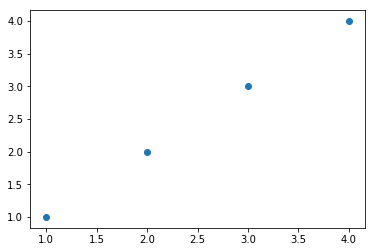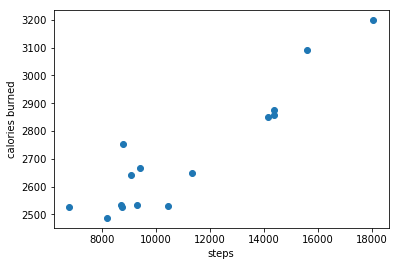Data Visualizations Matplotlib Plotting Tutorial

# Scatter Plots using Matplotlib

#### Import Modules¶

In :
import matplotlib.pyplot as plt
% matplotlib inline


#### What is Matplotlib¶

In Python, we utilize the module matplotlib to generate plots. We can create scatter plots, line plots, bar charts, histograms and more.

pyplot is an interface to matplotlib to provide easier syntax for plotting.

% matplotlib inline allows us to immediately see these plots inline in our Jupyter Notebook.

#### Generate a Simple Scatter Plot¶

We call the scatter function and must pass in at least two arguments, the first our list of x-coordinates, and the second our list of y-coordinates.

In :
plt.scatter([1, 2, 3, 4], [1, 2, 3, 4]);We plotted 4 scatter points.

#### Generate a Scatter Plot with My Fitbit Activity Data¶

Below is my Fitbit data of daily steps taken and daily calories burned over a 15-day period.

In :
calories_burned = [3092, 2754, 2852, 2527, 3199, 2640, 2874, 2649,
2525, 2858, 2530, 2535, 2487, 2534, 2668]

In :
steps = [15578, 8769, 14133, 8757, 18045, 9087, 14367, 11326, 6776,
14359, 10428, 9296, 8177, 8705, 9426]

In :
plt.scatter(steps, calories_burned)
plt.xlabel("steps")
plt.ylabel("calories burned");In order to more easily see the variation, by default, Matplotlib labels the smallest y-axis tick at 2500 instead of simply 0.

We can see a positive relationship between these two variables. The more steps I took in day, the more calories I burned.